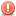CodeGuru Home VC++ / MFC / C++ .NET / C# Visual Basic VB Forums Developer.com

1.Junior MemberJoin Date
Jan 2009
Posts
1

##PHP MySQL problem

Hello guys,
I am having a search script which suppose to take results out of my mysql
database and display on a page 10 results each
if the results are more then 10 it should create another page
or pages with Next >> and Prev << link so anyone can move
forward and backward on those results page.

This script do everything fine counting total records display's 1 to 10
records on first page but at second location on same page i want it to daisplay
Showing results 1 to 10 of total records found it show 5 to 14, ant it
displays a link to Next which is not working.

Here is the code to generate pages please have a look and let me
know where I am wrong.

PHP Code:
``` // begin to show results setecho " found  \$numrows results <p>";\$count = 1 + \$s;// now you can display the results returned  while (\$row= mysql_fetch_array(\$result)) {  \$title = \$row["sname"];  \$url = \$row["url"];  \$message = \$row["massage"];//&nbsp;<a href=\"\$row['url']\">"\$row['sname']</a><br /> \$row['massage']<br><hr>'\  echo "\$count.)&nbsp";  echo "<b><a href=\"\$url\" target=\"_blank\">\$title</a></b>";  echo "<br>";  echo "\$message<br><hr height=12 color=lightblue><br>";  \$count++;  }\$currPage = ((\$s/\$limit) + 1);//break before paging  echo "<br />";  // next we need to do the links to other results &lt;&lt;  if (\$s>=1) { // bypass PREV link if s is 0  \$prevs=(\$s-\$limit);  print "&nbsp;<a href='{\$_SERVER['PHP_SELF']}?s=\$prevs&q=\$var'> <<<  Prev 10</a>&nbsp&nbsp;";  }// calculate number of pages needing links//  \$s=intval(\$numrows/\$limit);  \$s=ceil(\$numrows/\$limit);// \$s now contains int of pages needed unless there is a remainder from division  if (\$numrows%\$limit) {  // has remainder so add one page  \$s++;  }// check to see if last page  if (!(((\$s+\$limit)/\$limit)==\$s) && \$s!=1) {  // not last page so give NEXT link  \$PHP_SELF &gt;&gt;  \$news="\$s+\$limit";  echo "&nbsp;<a href='{\$_SERVER['PHP_SELF']}?s=\$news&q=\$var'>Next 10 >>> </a>";  }\$a = \$s+\$limit;  if (\$a > \$numrows) { \$a = \$numrows; }\$b = \$s+1;  echo "<p>Showing results \$b to \$a of \$numrows</p>";include ('footer.inc');?>  ```
Thank You
Last edited by PeejAvery; January 26th, 2009 at 06:55 PM. Reason: Added PHP tags.Reply With Quote

2. ## Re: PHP MySQL problem

Pagination is a very simple task. The following should get you through it.

PHP Code:
``` <?php\$show = 10;\$page = (isset(\$_GET['page'])) ? \$_GET['page'] : 1;\$page--; // because databases start at zero, humans start at one\$start = \$page * \$show;\$query = "SELECT * FROM blog ORDER BY date DESC LIMIT \$start, \$show";\$sql = mysql_query(\$query);\$rows = mysql_fetch_object(mysql_query("SELECT FOUND_ROWS() AS `rows`"))->rows;while (\$row = mysql_fetch_object(\$sql)) {  // do your outputting from the database here}if (\$page > 0) {echo '<a href="?page=' . \$page . '">&lt;&lt; Newer Posts</a>';}\$total = ceil(mysql_num_rows(mysql_query("SELECT id FROM blog")) / \$show);if (\$page < \$total - 1) {echo '<a href="?page=' . (\$page + 2) . '">Older Posts &gt;&gt;</a>';}?> ```
Last edited by PeejAvery; March 28th, 2012 at 05:29 PM. Reason: Fixed the row countReply With Quote

php to genrate pages####Posting Permissions

• You may not post new threads
• You may not post replies
• You may not post attachments
• You may not edit your posts
•